SimulRPi (0.1.0a1.dev1) is a Python library that partly fakes RPi.GPIO and simulates some I/O devices on a Raspberry Pi (RPi).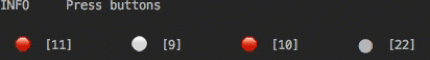Simulating LEDs on an RPi via a terminal

## Introduction¶

In addition to partly faking RPi.GPIO, SimulRPi also simulates these I/O devices connected to an RPi:

• push buttons by listening to pressed keyboard keys and

• LEDs by blinking dots in the terminal along with their GPIO pin numbers.

When a LED is turned on, it is shown as a red dot in the terminal. The pynput package is used to monitor the keyboard for any pressed key. Thus, the SimulRPi library can be useful in the case that you want to try your RPi.GPIO-based script by running it on your computer when no RPi is available at the moment.

Example: terminal outputSimulating LEDs on an RPi via a terminal

Each dot represents a blinking LED connected to an RPi and the number between brackets is the associated GPIO channel number. Here the LED on channel 22 toggles between on and off when a key is pressed.

Also, the color of the LEDs can be customized as you can see here where the LED on channel 22 is colored differently from the others.

Important

This library is not a Raspberry Pi emulator nor a complete mock-up of RPi.GPIO, only the most important functions that I needed for my Darth-Vader-RPi project were added.

If there is enough interest in this library, I will eventually mock more functions from RPi.GPIO.

## Dependencies¶

• Platforms: Linux, macOS

• Python: 3.5, 3.6, 3.7, 3.8

• `pynput` >=1.6.8: for monitoring the keyboard for any pressed key

## Installation instructions 😎¶

1. It is highly recommended to install `SimulRPi` in a virtual environment using for example `venv` or conda.

2. Make sure to update pip:

```\$ pip install --upgrade pip
```
3. Install the package `SimulRPi` (v0.1.0a1) with pip:

```\$ pip install SimulRPi
```

It will install the dependency `pynput` if it is not already found in your system.

Important

Make sure that pip is working with the correct Python version. It might be the case that pip is using Python 2.x You can find what Python version pip uses with the following:

```\$ pip -V
```

If pip is working with the wrong Python version, then try to use pip3 which works with Python 3.x

Note

To install the bleeding-edge version (0.1.0a1.dev1) of the `SimulRPi` package, install it from its github repository:

```\$ pip install git+https://github.com/raul23/SimulRPi#egg=SimulRPi
```

However, this latest version is not as stable as the one from PyPI but you get the latest features being implemented.

Warning message

If you get the warning message from pip that the `run_examples` script is not defined in your PATH:

```WARNING: The script run_examples is installed in '/home/pi/.local/bin' which is not on PATH.
```

Add the directory mentioned in the warning to your PATH by editing your configuration file (e.g. .bashrc). See this article on how to set PATH on Linux and macOS.

Test installation

Test your installation by importing `SimulRPi` and printing its version:

```\$ python -c "import SimulRPi; print(SimulRPi.__version__)"
```

## Usage¶

### Use the library in your own code¶

#### Case 1: with a `try` and `except` blocks¶

You can try importing `RPi.GPIO` first and if it is not found, then fallback on the `SimulRPi.GPIO` module.

Case 1: with a `try` and `except` blocks
```try:
import RPi.GPIO as GPIO
except ImportError:
import SimulRPi.GPIO as GPIO

```

The code from the previous example would be put at the beginning of your file with the other imports.

#### Case 2: with a simulation flag¶

Or maybe you have a flag to tell whether you want to work with the simulation module or the real one.

Case 2: with a simulation flag
```if simulation:
import SimulRPi.GPIO as GPIO
else:
import RPi.GPIO as GPIO

```

### Script `run_examples`¶

The `run_examples` script which you have access to once you install the `SimulRPi` package allows you to run different code examples on your RPi or computer. If it is run on your computer, it will make use of the `SimulRPi.GPIO` module which partly fakes `RPi.GPIO`.

The different code examples are those presented in Examples and show the capability of `SimulRPi.GPIO` for simulating I/O devices on an RPi such as push buttons and LEDs.

Here is a list of the functions that implement each code example:

#### List of options¶

To display the script’s list of options and their descriptions:

```\$ run_examples -h
```
-e

The number of the code example you want to run. It is required. (default: None)

-m

Set the numbering system (BCM or BOARD) used to identify the I/O pins on an RPi. (default: BCM)

-s

Enable simulation mode, i.e. `SimulRPi.GPIO` will be used for simulating `RPi.GPIO`. (default: False)

-l

The channel numbers to be used for LEDs. If an example only requires 1 channel, the first channel from the provided list will be used. (default: [9, 10, 11])

-b

The channel number to be used for a push button. The default value is channel 17 which is associated by default with the keyboard key cmd_r. (default: 17)

-k

The name of the key associated with the button channel. The name must be one of those recognized by the pynput package. See the SimulRPi documentation for a list of valid key names: https://bit.ly/2Pw1OBe. Example: alt, ctrl_r (default: cmd_r)

-t

Total time in seconds the LEDs will be blinking. (default: 4)

--on

Time in seconds the LEDs will stay turned ON at a time. (default: 1)

--off

Time in seconds the LEDs will stay turned OFF at a time. (default: 1)

-a

Use ASCII-based LED symbols. Useful if you are having problems displaying the default LED signs that make use of special characters. However, it is recommended to fix your display problems which might be caused by locale settings not set correctly. Check the article ‘Display problems’ @ https://bit.ly/35B8bfs for more info about solutions to display problems (default: False)

#### How to run the script¶

Once you install the `SimulRPi` package, you should have access to the `run_examples` script which can be called from the terminal by providing some arguments.

For example:

```\$ run_examples -e 1 -s
```

Let’s run the code example 5 which blinks a LED if a specified key is pressed:

```\$ run_examples -s -e 5 -l 22 -t 5 -k ctrl_r
```

Explanation of the previous command-line:

• `-s`: we run the code example as a simulation, i.e. on our computer instead of an RPi

• `-e 5`: we run code example 5 which blinks a LED if a key is pressed

• `-l 22`: we blink a LED on channel 22

• `-t 5`: we blink a LED for a total of 5 seconds

• `-k ctrl_r`: a LED is blinked if the key `ctrl_r` is pressed

Output: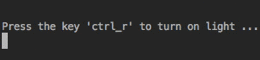Important

Don’t forget the -s flag when running the `run_examples` script as simulation, if you want to run a code example on your computer, and not on your RPi.

## Examples¶

The examples presented thereafter will show you how to use `SimulRPi` to simulate LEDs and push buttons.

The code for the examples shown here can be also found as a script in run_examples.

Note

Since we are showing how to use the `SimulRPi` package, the presented code examples are to be executed on your computer. However, the `run_examples` script which runs the following code examples can be executed on a Raspberry Pi or your computer.

### Example 1: display 1 LED¶

Example 1 consists in displaying one LED on the GPIO channel 10. Here is the code along with the output from the terminal:

```import SimulRPi.GPIO as GPIO

led_channel = 10
GPIO.setmode(GPIO.BCM)
GPIO.setup(led_channel, GPIO.OUT)
GPIO.output(led_channel, GPIO.HIGH)
GPIO.cleanup()
```

Output: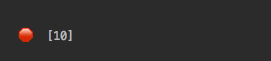The command line for reproducing the same results for example 1 with the `run_examples` script is the following:

```\$ run_examples -s -e 1 -l 10
```

Warning

Always call `cleanup()` at the end of your program to free up any resources such as stopping threads.

### Example 2: display 3 LEDs¶

Example 2 consists in displaying three LEDs on channels 9, 10, and 11, respectively. Here is the code along with the output from the terminal:

```import SimulRPi.GPIO as GPIO

led_channels = [9, 10, 11]
GPIO.setmode(GPIO.BCM)
GPIO.setup(led_channels, GPIO.OUT)
GPIO.output(led_channels, GPIO.HIGH)
GPIO.cleanup()
```

Output: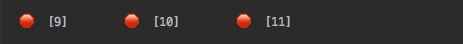The command line for reproducing the same results for example 2 with the `run_examples` script is the following:

```\$ run_examples -s -e 2
```

Note

In example 2, we could have also used a `for` loop to setup the output channels and set their states (but more cumbersome):

```import SimulRPi.GPIO as GPIO

led_channels = [9, 10, 11]
GPIO.setmode(GPIO.BCM)
for ch in led_channels:
GPIO.setup(ch, GPIO.OUT)
GPIO.output(ch, GPIO.HIGH)
GPIO.cleanup()
```

The `setup()` function accepts channel numbers as `int`, `list`, and `tuple`. Same with the `output()` function which also accepts channel numbers and output states as `int`, `list`, and `tuple`.

### Example 3: detect a pressed key¶

Example 3 consists in detecting if the key `cmd_r` is pressed and then printing a message. Here is the code along with the output from the terminal:

```import SimulRPi.GPIO as GPIO

channel = 17
GPIO.setmode(GPIO.BCM)
GPIO.setup(channel, GPIO.IN, pull_up_down=GPIO.PUD_UP)
print("Press key 'cmd_r' to exit\n")
while True:
if not GPIO.input(channel):
print("Key pressed!")
break
GPIO.cleanup()
```

Output: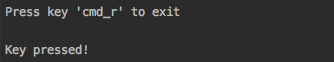The command line for reproducing the same results for example 3 with the `run_examples` script is the following:

```\$ run_examples -s -e 3 -k cmd_r
```

Note

By default, `SimulRPi` maps the key `cmd_r` to channel 17 as can be seen from the default key-to-channel map.

See also the documentation for SimulRPi.mapping where the default keymap is defined.

## How to uninstall 😞¶

To uninstall only the package `SimulRPi`:

```\$ pip uninstall simulrpi
```

To uninstall the package `SimulRPi` and its dependency:

```\$ pip uninstall simulrpi pynput
```